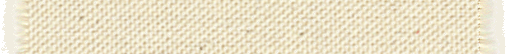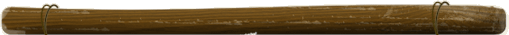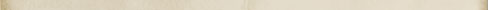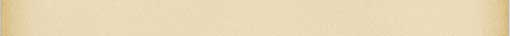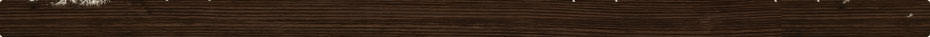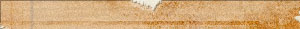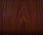# Layout Selection Language

In order to implement the layout selection we setup the QtScript instead of using our own scripting language since it's already working. At some point we'll want to switch to our own version for speed (i.e. pre-compiled byte code.)

The QtScript is plain JavaScript without any special extensions, however only the system will generate code so we're safe on that end.

The script is expected to return the name of the column defining the layout to use, or an empty string. If empty, then the process is repeated with the page type and its parents (see Layout feature [core]) This column name is later used to read the information about the layout, i.e. layout::data::<column-name>::layout_format. The column name could be "mobile" (for smart phones), "bare" (for lynx), "default" (for the rest.)

With a QtScript you define a variable and the last line references that variable. That will be the result of the script:

```var r;
r = plugins.content.modified > 145784 ? "this-name" : "that-name";
r;```

If the expression is simple enough, you may just enter the expression by itself:

`plugins.layout.name.toLowerCase() == "bare" ? "bare" : ""`

The plugins are accessible via the plugins variable followed by the name of the plugin followed by the name of the name of the field to retrieve. You may use the array syntax as well (plugins["content"]["modified"].) The type of these fields depends on the variable member that you retrieve. The fields are read-only from the JavaScript code.

All the normal JavaScript objects and operators are supported.

Note that this solution is currently slow because it has to build all those objects and that can take quite a bit of time... although we can reuse the same script environment over and over again (and avoid re-initializing everything) but that doesn't prevent the problem of having to recompile the JavaScript each time you connect to a snap_child object.

In order to implement the layout selection we created a small C like language that can compile expressions on the fly, so one can write expressions in that language, stick that expression in the layout system and the layout process executes those expressions and ends up with the advanced user selection.

The language allows for quite advanced selections but it is in itself very simple (especially if you know C, PHP, Java, JavaScript...) It supports many comparison operators in order to allow all sorts of comparions without the need to support functions.

For example, the following would select the Monday layout on Mondays:

`select "Monday" { layout::day_of_the_week == "Monday" };`

### Literals

Expressions can include literal

• Integers -- [0-9]+
• Floating points -- [0-9]+.[0-9]+(e[-+][0-9]+)?
• Booleans -- true and false
• Strings (written between double quotes: "this is a string".)

### Ranges

Ranges are composed of two values written between square brackets and separated by a colon as in:

`layout::hour *= [1:5];`

This expression returns true if the hour is between 1 and 5 (note that this would not be 1pm to 5pm, but 1am to 5am.)

### Arrays (also called Lists)

Arrays are lists of values. All the values in an array must have the same type. These values are kept sorted to facilitate comparisons between arrays. There is no operator to access the content of an array (the square brackets are reserved for ranges.)

The following is an example of an array of even integers from 0 to 10 checked against the number of comments in a page:

`content::comment_count &= { 0, 2, 4, 6, 8, 10 };`

The syntax is the same as in C/C++ arrays.

### Variables

Variables, or dynamic parameters, are defined by plugins that understand the layout signal layout_parameter which takes two parameters: the result (a reference to a QVariant,) and the name of the parameter (a QString).

The system supports the following variants:

• QString
• bool
• char, short, int, qlonglong, and corresponding unsigned variants
• float, double
• QList of any of those types of variants, but one list is expected to be composed of one type of variant (i.e. all QString's, all int's, etc.); these are considered to be arrays

Date and time are all expressed as the Unix time_t * 1000000 so we use the qlonglong for those numbers.

Days of the week and months for dates are expected to be numbers. This way it is easy to compare them (opposed to a name that can change in case and with the locale.) The day and month matches the POSIX struct tm definition: 0 is Sunday, 6 is Saturday; and the month goes from 0 (January) to 11 (December.)

URIs are returned as strings (Not QUrl).

### Operators

The supported operators are as follow:

Level Operator Description Grouping
1 :: scope Left-to-right
2 ~ ! + - unary (prefix) Right-to-left
3 ** power Right-to-left
4 * / % multiplicative Left-to-right
5 + - & additive Left-to-right
6 << >> >>> !< !> shift, rotate Left-to-right
7 <? >? minimum, maximum Left-to-right
8 < > <= <<= >= >>= ~= *= **= &= relational Left-to-right
9 == != === !== equality Left-to-right
10 && logical AND Left-to-right
11 ^^ logical XOR Left-to-right
12 || logical OR Left-to-right
13 ?: conditional Right-to-left

#### Scope

The scope operator is used to separate the name of a plugin from the name of the parameter to retrieve from that plugin (i.e. content::modified).

In effect this is the same as the name of the parameter in the database. The name of the plugin is used to access the plugin directly, something like this:

`param = get_plugin("content")->get_param("content::modified");`

#### Unary

The ~ can be used against integers in which case the bitwise not is applied. It can also be applied against strings to get all the characters in lowercase (useful to compare case insensitively.)

The ! can be used against booleans to reverse true to false and vice versa. Against a string, it returns true if the string is not empty, and false if the string is empty. Against integers and floating points it returns true if the value is not zero, and false otherwise. -0.0 is considered to be zero and thus returns false.

The + sign can be used against integers and floating points although it has no effect. It is here for completeness.

The - sign can be used against integers and floating points to negate those values.

These operators can be used against a list in which case all the elements of the list are affected.

#### Power

Compute the left hand side power the right hand side. Notice that this operator goes right to left. The power works with integers and floating points. Note that with integers you quickly get an overflow.

#### Multiplicative

Multiply (*), divide (/), and compute the modulo (%) of integers or floating points. As in C/C++ the result is a floating point if one of the operands is a floating point. All the computations are done with either qlonglong or double types.

Add (+) and subtract (-) integers or floating points. As in C/C++ the result is a floating point if one of the operands is a floating point. All the computations are done with either qlonglong or double types.

The concatenate (&) operator is used to concatenate two strings together. This operator is used instead of the common + in C++ to avoid the possibility of auto-converting strings to integers or floating points.

#### Shift, Rotate

The left shift (<<), right shift (>>), unsigned right shift (>>>), left rotate (!<) and right rotate (!>) can be applied to integers. The rotate is similar to shifting left and unsigned shifting right then merging the resulting bits, but it is done at once (read the parameter only once!)

#### Minimum, Maximum

All the values support the <? (minimum) and >? (maximum) operators. The left and right operands must be of the same type (which makes it easier to determine the type of the result.)

#### Relational and Equality

The relational can be used between two strings or between two numbers of any type (casting automatically happen as in C++ in that case.)

The relational can also be used against lists in which case each element is compared one after another and the expected result is returned. The elements are sorted so we will compare equal elements first and find the first pair of unequal elements and return that comparison's result. To be equal two lists must both have the exact same elements.

When comparing numbers, the result is as expected in C++. If a floating point is set to infinity or NaN, then the result may be undefined.

The ===, !==, <<=, and >>= operators are considered strict comparators. As such their result is false if the left hand side and right hand side variants are not of the exact same type (i.e. two shorts, two strings, two floats, etc.)

The <<= and >>= do not have a corresponding < and >. Instead use the not operator as in:

```!(... >>= ...)
!(... <<= ...)```

For strings, the ==, !=, <, <=, > and >= operators compare the strings ignoring case. Use the strict operators ===, !==, <<= and >>= to compare strings case sensitively.

The ~= operator is used to match the left hand side to the regular expression on the right hand side.

The *= operator is used to test whether a value (or at least one of the values in a list of values) is defined in a range. The range is defined as '[' <start-value> ':' <end-value> ']'. start-value is expected to be smaller or equal to <end-value>, if not the expression is considered invalid (i.e. we do not support empty ranges.)

Similarly, the **= operator is used to test whether a value is defined in a range. The semantic changes for lists: in this case each element of the list must individually be part of the range.

The &= operator is used to compute the intersection between the list on the left and the list on the right. It returns true if the intersection is not the empty set, false otherwise. The left hand side may be one element in which case it is viewed as a list of one element.

There is no &&= since it would be equivalent to ===.

#### Logical AND, XOR, and OR

Boolean values can be combined using these three operators. Be careful with the priority of each operator. If the left or right hand side is not an Boolean value, then the same is applied as with the logical not (!) operator.

#### Conditional

The conditional operator is like in C. The computes the expression on the left side of the question mark and if true it returns the result of the expression between the question mark and the colon, otherwise it returns the result of the expression after the colon.

It is common practice to write such expressions between parenthesis.

Since this language does not enforce types like C/C++, both results can be of different types although it is preferable to make it on the safe side by returning the exact same types on both sides.

#### Casting

The language does not include any explicit casting on purpose to simplify the syntax.

If you want to cast some values, you can use an expression as follow:

```To string: <expr> & ""
To floating point: <expr> * 1.0
To integer: (<expr> & "") + 0
To Boolean: !!<expr>```

However, in most cases it should not be necessary to do any casting.

It is unlikely that you would need to mask an integer, but if necessary, you can remove bits from an integer using the shift operators. Remember that qlonglong are used for such operators so you want to use 64 bits shifts:

`(((<expr> << 56) >> 60) << 4)`

is equivalent to the following in C

`<expr> & 0x0F0`

Note that you may not need to shift back by 4 and you may also be able to optimize using rotations.

### Grammar

The grammar includes the select keyword. This is the only keyword in this grammar.

```main: matches

matches: match
| match match

match: select STRING '{' conditional '}' ';'

conditional: logical_or '?' conditional : conditional
| logical_or

logical_or: logical_xor '||' logical_xor
| logical_xor

logical_xor: logical_and '^^' logical_and
| logical_and

logical_and: equality '&&' equality
| equality

equality: relational '==' relational
| relational '!=' relational
| relational '===' relational
| relational '!==' relational
| relational

relational: minmax '<' minmax
| minmax '<=' minmax
| minmax '<<=' minmax
| minmax '>' minmax
| minmax '>=' minmax
| minmax '>>=' minmax
| minmax '~=' minmax
| minmax '*=' minmax
| minmax '**=' minmax
| minmax '&=' minmax
| minmax

minmax: shift '<?' shift
| shift '>?' shift
| shift

| multiplicative '-' multiplicative
| multiplicative '&' multiplicative
| multiplicative

multiplicative: power '*' power
| power '/' power
| power '%' power
| power

power: unary '**' power
| unary

unary: '+' unary
| '-' unary
| '~' unary
| '!' unary
| primary

primary: STRING
| INTEGER
| FLOAT
| '[' minmax ':' minmax ']'
| '{' list '}'
| scoped_variable

list: minmax
| minmax ',' minmax

scoped_variable: IDENTIFIER '::' variable

variable: IDENTIFIER
| variable '::' IDENTIFIER
```Snap! Websites
An Open Source CMS System in C++